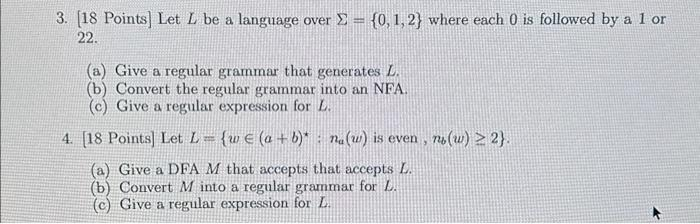Rating : ⭐⭐⭐⭐⭐
Price : \$10.99
Language:EN
Pages: 3
Words: 490

# Regular Grammar and NFA Homework Answers Needed

3. [18 Points] Let L be a language over Σ={0,1,2} where each 0 is followed by a 1 or 22. (a) Give a regular grammar that generates L. (b) Convert the regular grammar into an NFA. (c) Give a regular expression for L. 4. [18 Points ] Let L={w∈(a+b)⋆:na​(w) is even, nb​(w)≥2}. (a) Give a DFA M that accepts that accepts L. (b) Convert M into a regular grammar for L. (c) Give a regular expression for L.# Step By Step Answers with Explanation

### Question 3:

1. S → 0A

2. A → 1A | 22A | ε

• States: {S, A, Accept}

1. Define transitions based on the productions:

• A → 22A

• Transition: A --(2)--> A

(c) Regular Expression for Language L:

To find a regular expression for L, we can start from the NFA and apply the standard procedures for converting an NFA to a regular expression. The regular expression for L is quite complex, so I'll provide it step by step:

• A --(2)--> A

• A --(ε)--> Accept

1. Finally, we add the transition from S to A and wrap the entire expression with brackets:

• (0(A --(1)--> A | A --(2)--> A)*) | ε

### Question 4:

• Define states:

1. q0: Initial state

• q0 --(a)--> q1

• q0 --(b)--> q2

• q3 --(a)--> q1

• q3 --(b)--> q2

1. Start symbol: S

2. Productions:

(c) Regular Expression for Language L:

To find a regular expression for L, we can start with the DFA and apply the standard procedures for converting a DFA to a regular expression. The regular expression for L is:

How It Works